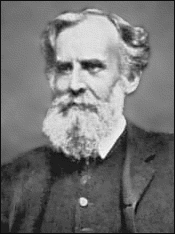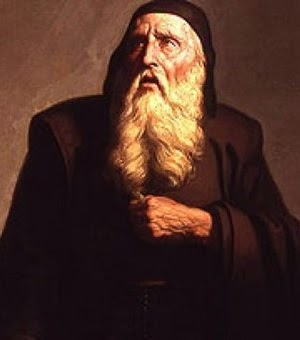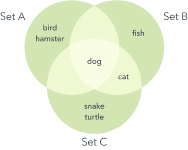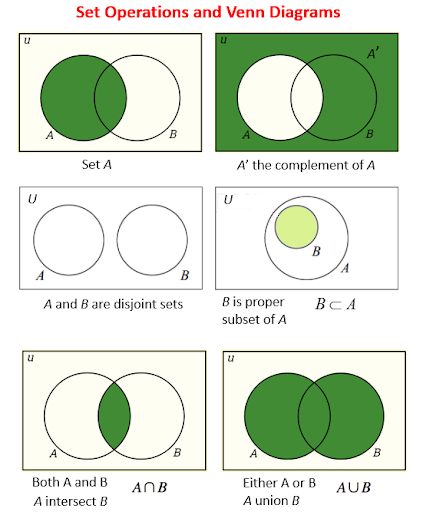Math Concepts

# What is a Venn Diagram?

151 views

Table of Contents

 1 Introduction 2 Understanding the Venn Diagram 3 Venn diagram history 4 Applications for Venn Diagrams 5 Example Venn diagram 6 Venn diagram purpose and benefits 7 Practice Example 8 Frequently Asked Questions (FAQs) 9 External References

29 October  2020

Reading Time: 6 Minutes

A Venn diagram is an illustration that uses circles to show the relationships among things or finite groups of items. Circles that overlap have a commonality, while circles that do not overlap do not share those traits.

Venn diagrams help to visually represent the similarities and differences between the two concepts. They have long been recognized for their usefulness as educational tools.

Since the mid-20th century, Venn diagrams have been used as part of the introductory logic curriculum and in elementary-level educational plans around the world.

Since the mathematical term for "a group of things" is "a set", Venn diagrams can be used to illustrate set relationships. Download the PDF to understand Venn diagrams in detail.

 📥 Understanding the Venn Diagram Download

Also read:

## Understanding the Venn Diagram

The English logician John Venn popularized the diagram in the 1880s. He called them Eulerian circles after the Swiss mathematician Leonard Euler, who created similar diagrams in the 1700s.

The term Venn diagram did not appear until 1918 when Clarence Lewis, an American academic philosopher and the eventual founder of conceptual pragmatism, referred to the circular depiction as the Venn diagram in his book A Survey of Symbolic Logic.

Venn diagrams have been used since the mid-20th century in classrooms from the elementary school level to introductory logic.

Venn studied and taught logic and probability theory at Cambridge University, where he developed his method of using diagrams to illustrate the branch of mathematics known as set theory.

Venn published a precedent-setting work, The Logic of Chance, which explained the frequency theory of probability. He argued that probability, contrary to widespread assumption, should be established based on the regularity with which something is predicted to occur.

In another book, Symbolic Logic, he built on and developed mathematician George Boole’s theories on algebra. This work helped him develop the Venn diagram.

## Venn diagram history

Venn diagrams are named after British logician John Venn. He wrote about them in an 1880 paper entitled “On the Diagrammatic and Mechanical Representation of Propositions and Reasonings” in the Philosophical Magazine and Journal of Science.But the roots of this type of diagram go back much further, at least 600 years. In the 1200s, philosopher and logician Ramon Llull (sometimes spelled Lull) of Majorca used a similar type of diagram, wrote author M.E. Baron in a 1969 article tracing their history.

She also credited German mathematician and philosopher Gottfried Wilhelm von Leibnitz with drawing similar diagrams in the late 1600s.In the 1700s, Swiss mathematician Leonard Euler (pronounced Oy-ler) invented what came to be known as the Euler Diagram, the most direct forerunner of the Venn Diagram. John Venn referred to his diagrams as Eulerian Circles, not Venn Diagrams.

The term Venn Diagrams was first published by American philosopher Clarence Irving (C.I.) Lewis, in his 1918 book, A Survey of Symbolic Logic.

Venn Diagrams continued to evolve over the past 60 years with advances by experts David W. Henderson, Peter Hamburger, Jerrold Griggs, Charles E. “Chip” Killian, and Carla D. Savage.

Their work concerned symmetric Venn Diagrams and their relationship to prime numbers, or numbers indivisible by other numbers except 1 and the number itself. One such symmetric diagram, based on prime number 7, is widely known in math circles as Victoria.

Other notable names in the development of Venn Diagrams are A.W.F. Edwards, Branko  Grunbaum, and Henry John Stephen Smith. They changed the shapes in the diagrams to allow simpler depiction of Venn Diagrams at increasing numbers of sets.

## Applications for Venn Diagrams

Venn diagrams depict how items relate to each other against an overall backdrop, universe, data set, or environment.

A Venn diagram could be used, for example, to compare two companies within the same industry by illustrating the products both companies offer (where circles overlap) and the products that are exclusive to each company (outer circles).

At a basic level, Venn diagrams are simple pictorial representations of the relationship between two sets of things. However, they can be much more complex.

Still, the Venn diagram’s streamlined purpose to illustrate concepts and groups has led to their popularized use in many fields, including statistics, linguistics, logic, education, computer science, and business.

## Example of Venn diagram

Say our universe is pets, and we want to compare which type of pet our family might agree on

Set A contains my preferences: dog, bird, hamster.

Set B contains Family Member B’s preferences: dog, cat, fish.

Set C contains Family Member C’s preferences: dog, cat, turtle, snake.

The overlap, or intersection, of the three sets, contains only dog. Looks like we’re getting a dog.

Of course, Venn diagrams can get a lot more involved than that, as they are used extensively in various fields.## What are the purpose and benefits of Venn diagram?

• Students and professionals can use them to think through the logic behind a concept and to depict the relationships for visual communication. This purpose can range from elementary to highly advanced.
• To compare two or more choices and clearly see what they have in common versus what might distinguish them. This might be done for selecting an important product or service to buy.
• To solve complex mathematical problems. Assuming you’re a mathematician, of course.
• To compare data sets, find correlations, and predict probabilities of certain occurrences.

The following diagrams show the set operations and Venn Diagrams for Complement of a Set, Disjoint Sets, Subsets, Intersection, and Union of Sets. Scroll down the page for more examples and solutions.The set of all elements being considered is called the Universal Set (U) and is represented by a rectangle.

• The complement of A, A', is the set of elements in U but not in A. A' ={x | x ∈ U and x ∉ A}
• Sets A and B are disjoint sets if they do not share any common elements.
• B is a proper subset of A. This means B is a subset of A, but B ≠ A.
• The intersection of A and B is the set of elements in both set A and set B. A ∩ B = {x | x ∈ A and x ∈ B}
• The union of A and B is the set of elements in set A or set B. A ∪ B = {x | x ∈ A or x ∈ B}
• A ∩ ∅ = ∅
• A ∪ ∅ = A

## Practice Example

 Example 1

Given the set, P is the set of even numbers between 15 and 25. Draw and label a Venn diagram to represent the set P and indicate all P’s elements in the Venn diagram.

### Solution:

List out the elements of P.

P = {16, 18, 20, 22, 24} ← ‘between’ does not include 15 and 25

Draw a circle or oval. Label it P. Put the elements in P.Example 2

Draw and label a Venn diagram to represent the set

R = {Monday, Tuesday, Wednesday}.

### Solution:

Draw a circle or oval. Label it R. Put the elements in R.Example 3

Given the set Q = { x : 2x – 3 < 11, x is a positive integer }. Draw and label a Venn diagram to represent the set Q.

### Solution:

Since an equation is given, we need to first solve for x.

2x – 3 < 11 ⇒ 2x < 14 ⇒ x < 7So, Q = {1, 2, 3, 4, 5, 6}

Draw a circle or oval. Label it Q.

Put the elements in Q.

## Conclusion

Did you realize the importance of Venn diagrams?

• To compare two or more choices and clearly see what they have in common versus what might distinguish them.
• To solve complex mathematical problems.
• To visually organize information to see the relationship between sets of items, such as commonalities and differences.
• To compare data sets, find correlations, and predict probabilities of certain occurrences.
• To reason through the logic behind statements or equations, such as the Boolean logic behind a word search involving “or” and “and” statements and how they’re grouped.

## About Cuemath

Cuemath, a student-friendly mathematics and coding platform, conducts regular Online Live Classes for academics and skill-development, and their Mental Math App, on both iOS and Android, is a one-stop solution for kids to develop multiple skills. Understand the Cuemath Fee structure and sign up for a free trial.

## Frequently Asked Questions (FAQs)

### What is a Venn Diagram?

A Venn diagram is a pictorial representation of sets. It is used to represent the relationship between two or more sets. It shows a universal set, where all its subsets are displayed based on the element of sets.

### What is the history behind the invention of Venn diagrams?

Venn diagrams are named after British logician John Venn. In the 1700s, Swiss mathematician Leonard Euler invented what came to be known as the Euler Diagram, the most direct forerunner of the Venn Diagram. In fact, this is the reason John Venn referred to his diagrams as Eulerian Circles, not Venn Diagrams.

### What are the operations of sets that can be represented by Venn Diagrams?

A Venn diagram can represent the Union of sets, Intersection of sets, Complement of set, Difference between two sets.

### If a Venn diagram shows two circles which do not touch each other, then what does it mean?

In a Venn diagram, if the two circles show two independent sets and do not touch each other at any point, then they are said to be disjoint sets.

### What is the complement of complement of a set?

If A is a set, then its complement will be A’ and complement of the complement of a set A will be (A’)’ = A.

## External References

Related Articles
GIVE YOUR CHILD THE CUEMATH EDGE
Access Personalised Math learning through interactive worksheets, gamified concepts and grade-wise courses
Learn More About Cuemath